Test: Electrical & Electronic Measurements- 1

# Test: Electrical & Electronic Measurements- 1

Test Description

## 10 Questions MCQ Test GATE Electrical Engineering (EE) 2023 Mock Test Series | Test: Electrical & Electronic Measurements- 1

Test: Electrical & Electronic Measurements- 1 for Electrical Engineering (EE) 2023 is part of GATE Electrical Engineering (EE) 2023 Mock Test Series preparation. The Test: Electrical & Electronic Measurements- 1 questions and answers have been prepared according to the Electrical Engineering (EE) exam syllabus.The Test: Electrical & Electronic Measurements- 1 MCQs are made for Electrical Engineering (EE) 2023 Exam. Find important definitions, questions, notes, meanings, examples, exercises, MCQs and online tests for Test: Electrical & Electronic Measurements- 1 below.
Solutions of Test: Electrical & Electronic Measurements- 1 questions in English are available as part of our GATE Electrical Engineering (EE) 2023 Mock Test Series for Electrical Engineering (EE) & Test: Electrical & Electronic Measurements- 1 solutions in Hindi for GATE Electrical Engineering (EE) 2023 Mock Test Series course. Download more important topics, notes, lectures and mock test series for Electrical Engineering (EE) Exam by signing up for free. Attempt Test: Electrical & Electronic Measurements- 1 | 10 questions in 30 minutes | Mock test for Electrical Engineering (EE) preparation | Free important questions MCQ to study GATE Electrical Engineering (EE) 2023 Mock Test Series for Electrical Engineering (EE) Exam | Download free PDF with solutions
 1 Crore+ students have signed up on EduRev. Have you?
Test: Electrical & Electronic Measurements- 1 - Question 1

### The resistance of the circuit is found by measuring current flowing and power fed into the circuit. If limiting errors in the measurement of power and current are ± 2.5% and ± 1.5%. Respectively, the limiting error in the measurement of resistance will be _______ (in percentage)

Detailed Solution for Test: Electrical & Electronic Measurements- 1 - Question 1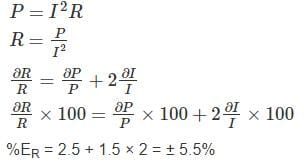Test: Electrical & Electronic Measurements- 1 - Question 2

### The battery voltage of an analog multimeter drops by 20% of its original value. The multimeter is used to measure dc voltage of a circuit. The true voltage compared to the measured value is

Detailed Solution for Test: Electrical & Electronic Measurements- 1 - Question 2

The internal battery of an analog multimeter is used to find resistance. The battery voltage doesn’t influence the measurement of voltage. Hence, the true value and measured value both are same.

*Answer can only contain numeric values
Test: Electrical & Electronic Measurements- 1 - Question 3

### A 50 V range voltmeter has a sensitivity of 40 KΩ/V. The total resistance of the voltmeter is – (in MΩ)

Detailed Solution for Test: Electrical & Electronic Measurements- 1 - Question 3

Given that,
Voltage range = 50 V
Sensitivity = 40 KΩ/V
Total resistance = Sensitivity × Voltage range
= 40 KΩ/V × 50 V
= 2000 KΩ
= 2 MΩ

*Answer can only contain numeric values
Test: Electrical & Electronic Measurements- 1 - Question 4

A voltmeter has 100 scale divisions and can measure up to 200 V. Each division can be read up to 1/5 division. The resolution of the voltmeter is – (in V)

Detailed Solution for Test: Electrical & Electronic Measurements- 1 - Question 4

100 divisions = 200 V
1 divisions = 2 V
1/5 division = 0.4 V
Resolution = 0.4 V

Test: Electrical & Electronic Measurements- 1 - Question 5

A resistance is determined by voltmeter ammeter method. The voltmeter reads 100 V with a probable error of ±12 V and ammeter reads 10 A with a probable error of ±2 A. Determine the probable error in the computed value of resistance

Detailed Solution for Test: Electrical & Electronic Measurements- 1 - Question 5

We have resistance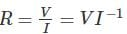Weighted probable error in the resistance due to voltage is,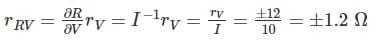Weighted probable error in the resistance due to current is,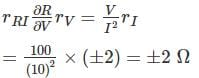Probable error in computed resistance is,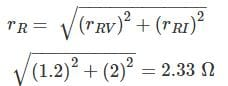*Answer can only contain numeric values
Test: Electrical & Electronic Measurements- 1 - Question 6

A voltage reading is to be taken across the load Resistor R­L in the circuit shown below. A voltmeter with a sensitivity 10 kΩ/V and a guaranteed accuracy of ± 5 % at full scale is to be used on its 20 V range. The maximum voltmeter reding that could be expected is __ (in V)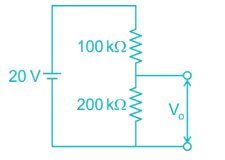Detailed Solution for Test: Electrical & Electronic Measurements- 1 - Question 6

Sensitivity of voltmeter = 10 kΩ/V
Full scale reading (VFSD) = 20 V
Internal resistance of voltmeter (Rv) = 10 kΩ/V × 20 V
= 200 kΩ
By voltage division,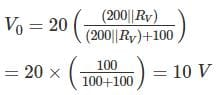Guaranteed accuracy of meter = ± 5% at FSD.
V0 = 10 ± (5% of 20) = 10 ± 1 = 9 to 11
Maximum voltmeter reading = 11 V.

*Answer can only contain numeric values
Test: Electrical & Electronic Measurements- 1 - Question 7

The resistance is measured by the voltmeter ammeter method the voltmeter reding is 50 V on 100 V scale and ammeter reading is 50 mA on 100 mA scale. If both the meters are guaranteed for accuracy with 2% of full scale, what is the limit within which resistance (in Ω ) can be measure?

Detailed Solution for Test: Electrical & Electronic Measurements- 1 - Question 7

V = 50 ± (2% of 100 V)
Im = 50 × 10-3 ± (2% of 100 mA)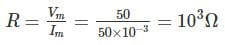Rm = 103 ± 4% = 1000 ± 40
= 960 Ω to 1040 Ω
Limit = 80 Ω

Test: Electrical & Electronic Measurements- 1 - Question 8

A 150 ± 10% Ω resistor is connected to the terminals of a power supply operating at 200 ± 0V. If the resistor varies over the range ± 10% of its expected value, what is the range of error in this current.

Detailed Solution for Test: Electrical & Electronic Measurements- 1 - Question 8

Given voltage range, V = 200 ± 0 V
Given resistor range, R = 150 ± 10% Ω
= [150 – 10%, 150 + 10%]
= [150 – 15, 150 + 15]
= [135, 165] Ω
When R = 135 Ω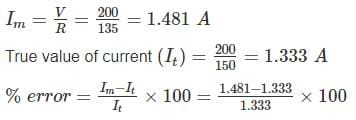= 11.13%
When R = 165 Ω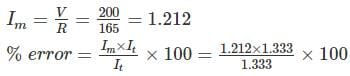= -9.07%
Range of error in the current = -9.07% to 11.13%

Test: Electrical & Electronic Measurements- 1 - Question 9

Which one of the following statement is not correct?

Detailed Solution for Test: Electrical & Electronic Measurements- 1 - Question 9

It is possible to have precise measurements which are not accurate. we can get precise results which may not accurate.
So option (a) is not correct

Test: Electrical & Electronic Measurements- 1 - Question 10

The current I flowing through a resistance of value 100 Ω ± 0.2% is I = 4A ± 0.5% the uncertainty in measurement of power is

Detailed Solution for Test: Electrical & Electronic Measurements- 1 - Question 10

When many variables are in valued is done on the same basis as is done for error analysis when the result are expressed as standard deviation or probable errors.
Let x = F(x1, x2, x3 …..)
Wx1, Wx2, …. Wxn be the uncertainties of x1, x2, … xn then uncertainty of x is given by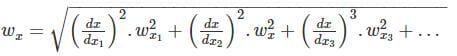Calculation:
R = 100 ± 0.2% = 100 ± 0.2
I = 4 ± 0.5% = 4 ± 0.2
P = 100 × 42 = 1600 W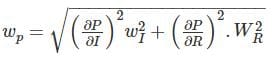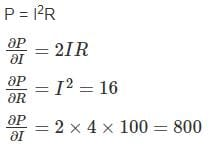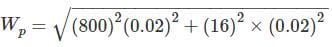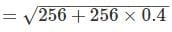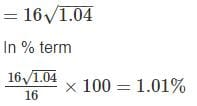## GATE Electrical Engineering (EE) 2023 Mock Test Series

22 docs|274 tests
Information about Test: Electrical & Electronic Measurements- 1 Page
In this test you can find the Exam questions for Test: Electrical & Electronic Measurements- 1 solved & explained in the simplest way possible. Besides giving Questions and answers for Test: Electrical & Electronic Measurements- 1, EduRev gives you an ample number of Online tests for practice

## GATE Electrical Engineering (EE) 2023 Mock Test Series

22 docs|274 tests(Scan QR code)